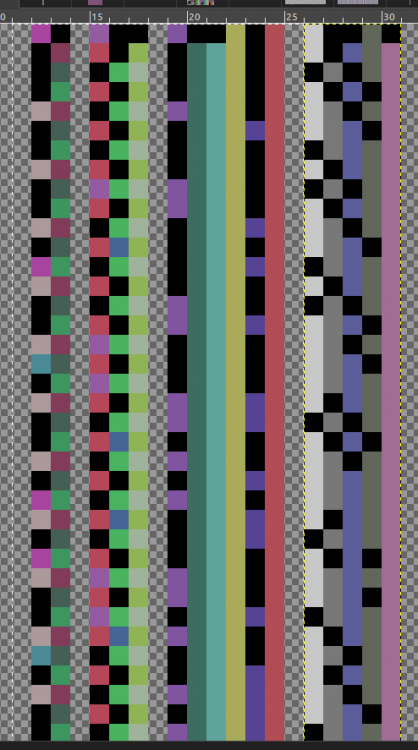## Recommended Posts

For any prime number n > 3, n mod 6 = 1 or 5. any prime number n > 3, n mod 3 = 1 or 2. The same prime numbers are in column 1 either way and the primes from column 2 (mod 3) are in column 5 (mod 6).
Are there 2 kinds of prime numbers? Is there a name for these primes?

The attached png is the primes mod 2,3,6, and 7.##### Share on other sites

38 minutes ago, moth said:

For any prime number n > 3, n mod 6 = 1 or 5. any prime number n > 3, n mod 3 = 1 or 2. The same prime numbers are in column 1 either way and the primes from column 2 (mod 3) are in column 5 (mod 6).
Are there 2 kinds of prime numbers? Is there a name for these primes?

I don't think they have names, but there's a famous theorem of Fermat that says an odd prime is the sum of two squares if and only if p = 1 (mod 4).

##### Share on other sites

Thanks for the link. Found some good stuff in the "see also" section too. Now I think I'm misusing the term "Mod". The '%' operator in C is the remainder from integer division, is the result of that operation the same as the mod operation ?

##### Share on other sites

58 minutes ago, moth said:

Thanks for the link. Found some good stuff in the "see also" section too. Now I think I'm misusing the term "Mod". The '%' operator in C is the remainder from integer division, is the result of that operation the same as the mod operation ?

Great question. Yes they are "the same but a little different."

In math, mod is an equivalence relation on the integers. We say that $a \equiv b \pmod n$ for two integers $a, b$ if it happens to be the case that $n | a - b$, where the vertical bar means "divides." So for example $5 \equiv 3 \pmod 2$ and $17 \equiv 5 \mod 3$.

You can verify that this is an equivalence relation: It's reflexive, symmetric, and transitive. It partitions the integers into $n$ pairwise-disjoint equivalence classes. It's a fundamental concept in number theory.

In programming languages, mod is a binary operator that inputs two integers and outputs a third: 5 % 3 = 2. It inputs 5 and 3, and outputs the remainder when 5 is integer-divided by 3.

The math and programming concepts are closely related. $a % n$ is the remainder when $a$ is integer-divided by $n$; that is, the result of $a % n$ is the smallest positive element of the equivalence class of $a$ under the $\pmod n$ equivalence relation. This turns out to be the same as the remainder under integer division.

The tl;dr is that in math, mod is an equivalence relation that inputs a pair of numbers and a modulus and returns True or False; whereas in programming, mod is a binary operator that inputs an integer and a modulus and outputs the smallest positive member of the equivalence class of the integer mod the modulus.

The difference is shown by, say, the fact that $17 \equiv 14 \pmod 3$; but $17 \ \% \ 3 = 2$ and not $14$.

That is. even though mathematically $17 \equiv 14 \pmod 3$, in a programming language, $17 \ \% \ 3 == 14$ would evaluate to False. That's an example that illustrates the difference.

Edited by wtf
##### Share on other sites

I think I see the difference now. 5 and 3 (and all odd numbers?) are equivalent mod 2 so the mod operator returns 'true' while the '%' operator returns the same value (1) for (any odd number) mod 2, but the '%' operator would take a few iterations to determine if two integers are in the same equivalence set?

##### Share on other sites

51 minutes ago, moth said:

I think I see the difference now. 5 and 3 (and all odd numbers?) are equivalent mod 2 so the mod operator returns 'true' while the '%' operator returns the same value (1) for (any odd number) mod 2,

Yes exactly.

51 minutes ago, moth said:

but the '%' operator would take a few iterations to determine if two integers are in the same equivalence set?

I suppose you'd have to subtract the two integers and see if the result is integer-divisible by the modulus. That seems like the most sensible way. Probably not the only way.

##### Share on other sites

Thanks for clearing that up. @wtf.  Reading the Wikipedia page about equivalence classes now. In the pdf i attached, each column is an equivalence class for mod 2, 3, 6, and 5.

## Create an account or sign in to comment

You need to be a member in order to leave a comment

## Create an account

Sign up for a new account in our community. It's easy!

Register a new account